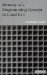# Exercises5.1 What is the difference between invocation and activation of a function?

5.2 Where is storage for the "auto" variables located?

5.3 Does every local variable have the "auto" storage class?

5.4 Explain why the variables of a function f() cannot retain values between two consecutive activations of f() .

5.5 What are the differences between the system stack and the system heap? Try to list all differences - in their roles and purpose as well as in their implementations .

5.6 Define the following terms: calling sequence, calling convention, return sequence, flow of control. How are they related to execution of functions?

5.7 If a local variable is defined as static , does this make it global?

5.8 Is there any difference between C and C++ with regard to calling functions and passing arguments to them?

5.9 Which of the calling methods discussed in this chapter are relevant for C? Which are relevant for C++?

5.10 Rewrite the following simple C function, which receives an integer parameter, so that it emulates receiving the parameter by "reference".

` void doit(int x)    {      printf("%d\n",x);      x++;      printf("%d\n",x);    } `

5.11 Rewrite the recursive descent parser used in this chapter to display (in a schematic fashion) what the system stack "looks like" during the execution: when a function is entered, display the "new activation frame"; when the function is exited, display the "previous activation frame". Use indentation to indicate how the stack grows (moving the indentation to the right) and how it shrinks (moving the indentation to the left). Then execute the program with input string "..a.." . Compare your result with the diagrams shown here.

5.12 Repeat Exercise 5.11 for the Hanoi towers puzzle program listed in Appendix A.

5.13 Rewrite the recursive descent parser used in this chapter so that s and sp are global variables.

5.14 The following recursive program generates and displays all bit combinations for a byte.

` #include <stdio.h>    char byte;    void bits(int n,char byte[])    {     int i;     if (n == 8) {       for(i = 0; i < 8; i++)        putchar(byte[i]);       putchar('\n');       return;     }      byte[n] = '0';      bits(n+1,byte);      byte[n] = '1';      bits(n+1,byte);    }    int main()    {      bits(0,byte);      return 0;    } `

We now rewrite it using global variables instead of arguments being passed along. Will it work? Justify your answer.

` #include <stdio.h>    char byte;    int n;    void bits()    {     int i;     if (n == 8) {       for(i = 0; i < 8; i++)        putchar(byte[i]);       putchar('\n');       return;     }      byte[n] = '0';      n = n+1;      bits();      n = n-1;      byte[n] = '1';      n = n+1;      bits();    }    int main()    {      n=0;      bits();      return 0;    } `Memory as a Programming Concept in C and C++
ISBN: 0521520436
EAN: 2147483647
Year: 2003
Pages: 64

Similar book on Amazon

flylib.com © 2008-2017.
If you may any questions please contact us: flylib@qtcs.net